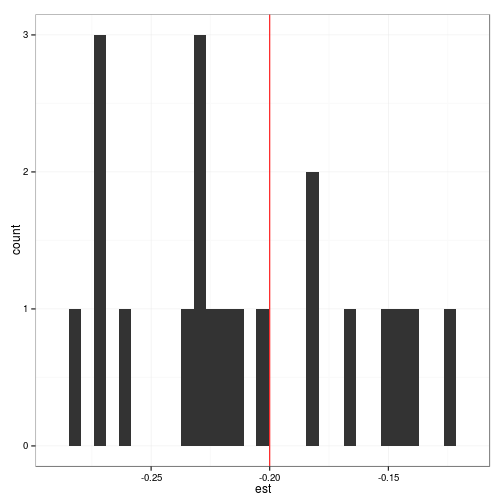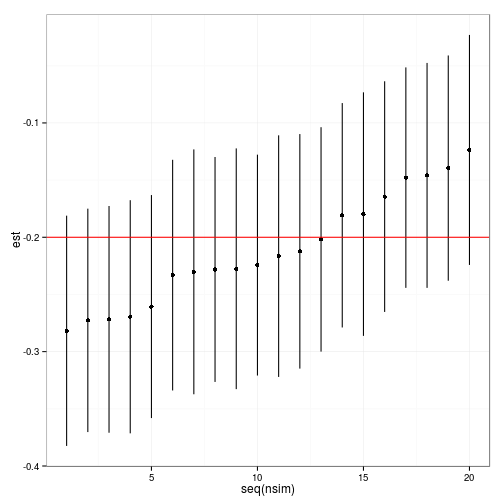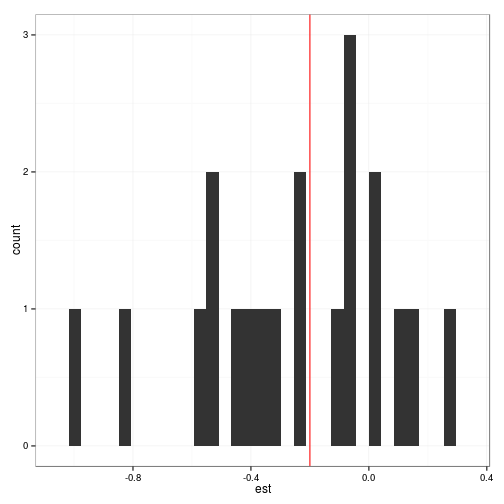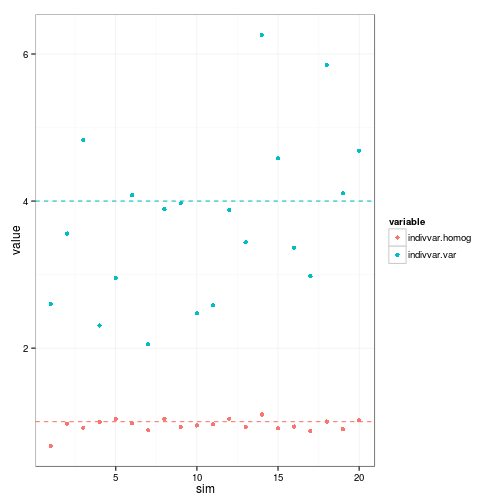# Simulation-based power analysis for mixed models in `lme4`

Power analysis of mixed models often has to be based on simulation because the required analytical tools for calculating the sampling distributions in situations where the null hypothesis is false (or even in those where it is true) is lacking. There are a variety of existing R packages built on `nlme` and `lme4` that tackle this problem (`pamm`, `longpower`, `clusterPower`, `odprism`, `nlmeU`), but here I wanted to show a more flexible approach based on the recently introduced simulation capabilities of the `lme4` package. This allows power calculations for GLMMs, for example, although it does not allow power calculations for problems with “R-side” effects (temporal and spatial autocorrelation, heteroscedasticity) which cannot currently be handled in `lme4`.

You will neeed a fairly recent/development version of `lme4`; if you have compilation tools installed, the easiest way to get the very latest version is:

``````library(devtools)
install_github("lme4", user = "lme4")
``````

You can also try your luck with:

``````install.packages("lme4", repos = c("http://lme4.r-forge.r-project.org/repos",
getOption("repos")))
``````

(although you will have to see whether the version you get that way is sufficiently up to date: check `?simulate.merMod` and see whether it mentions a `formula` argument or not).

``````library(lme4)
``````
``````## Loading required package: Matrix
``````
``````packageVersion("lme4")
``````
``````##  '1.1.3'
``````
``````library(plyr)
library(ggplot2)
theme_set(theme_bw())
``````

The general recipe is as follows:

• Set up a data frame containing information about the experimental design (number of treatments, number of individuals per treatment, number of observations per individual): `expand.grid()` is useful. If you have any continuous predictors or covariates, then you need to figure out what the distribution of that is going to be: is it regular/linear, or Normally distributed, or uniform? If you want to include observation-level random effects/overdispersion, then add an `obs` variable to the data frame which is defined simply as `factor(seq(nrow(your_data)))`.
• set up the formula corresponding to the model you're going to want to fit: in the simple case where you assume that the among-individual variation is the same across treatments, something like `proprange~treat+(1|indiv)+(1|obs)` (this formula includes observation-level variation to account for overdispersion in the binomial)
• specify the parameters: `"theta"` - in the case of single variance terms, that's just the standard deviation (on the logit scale) of each random effect: e.g. `theta=c(1,0.5)` (among-individual variation is 4x among-observation variance). `"beta"` is the fixed-effects parameters, in this case `(intercept,treat)` – also all on the logit scale. For LMMs you should also specify `"sigma"`, the residual standard error.
• simulate on this basis: `simulate(formula,newdata,parameters,family=binomial)` (or something) – returns a response vector. See `?simulate.merMod` for an example.
• run the `glmer` and extract p-values or whatever
• do this with a range of numbers of individuals per treatment, obs per individual, etc..

### Example

Two treatments, 30 individuals per treatment, 5 observations per individual.

``````expdat <- expand.grid(indiv = factor(1:30), obs = factor(1:5), ttt = c("homog",
"var"))
expdat\$obs <- factor(seq(nrow(expdat)))
``````

Parameters: baseline range (fraction of grid cells sampled) is 70% (logit(0.7)=0.8473), effect of treatment is to decrease log-odds of range by 0.4 (approx 0.4/4 = 10%). Random effects standard deviations of individual and observation are both 1.0 (on the same scale as the fixed-effect parameters).

``````set.seed(101)
nsim <- 20
beta <- c(qlogis(0.7), -0.2)
theta <- c(0.1, 0.1)
``````
``````ss <- simulate(~ttt + (1 | indiv) + (1 | obs), nsim = nsim, family = binomial,
weights = rep(25, nrow(expdat)), newdata = expdat, newparams = list(theta = theta,
beta = beta))
expdat\$resp <- ss[, 1]
fit1 <- glmer(resp ~ ttt + (1 | indiv) + (1 | obs), family = binomial, weights = rep(25,
nrow(expdat)), data = expdat)
``````
``````## Warning: Model failed to converge with max|grad| = 0.00187101 (tol =
## 0.001)
``````
``````fit1B <- refit(fit1, ss[])
fitsim <- function(i) {
coef(summary(refit(fit1, ss[[i]])))["tttvar", ]
}
``````
``````t1 <- system.time(fitAll <- laply(seq(nsim), function(i) fitsim(i)))
## you can use .progress='text' to get a progress indicator ...
fitAll <- setNames(as.data.frame(fitAll), c("est", "stderr", "zval", "pval"))
``````

(This took 0.3 minutes on my laptop.)

``````head(fitAll)
``````
``````##       est  stderr   zval      pval
## 1 -0.1807 0.05006 -3.611 3.051e-04
## 2 -0.1478 0.04919 -3.005 2.653e-03
## 3 -0.2606 0.04976 -5.237 1.633e-07
## 4 -0.2718 0.05057 -5.374 7.697e-08
## 5 -0.2695 0.05201 -5.181 2.202e-07
## 6 -0.2165 0.05391 -4.015 5.934e-05
``````

Power calculation:

``````with(fitAll, mean(pval < 0.05))
``````
``````##  1
``````

Estimates:

``````ggplot(fitAll, aes(x = est)) + geom_histogram() + geom_vline(xintercept = -0.2,
colour = "red")
``````Or

``````ggplot(arrange(fitAll, est), aes(x = seq(nsim), y = est, ymin = est - 1.96 *
stderr, ymax = est + 1.96 * stderr)) + geom_pointrange() + geom_hline(yintercept = -0.2,
colour = "red")
``````Once I have my simulation/estimation procedure working for a particular set of parameters, I then embed it in a large, nested `for` loop that explores the whole range of experimental design parameters I'm interested in (e.g. effect size, variance, number of blocks, samples per block, etc.). I generally find it most convenient to store everything in a large multi-dimensional array, with one dimension for each experimental design variable, a dimension for replicates, and as many dimensions as necessary for the information I want to save about each replicate. For example, if I was considering 10 possible numbers of samples per block, 10 possible numbers of blocks, and 5 possible effect sizes, doing 100 replicates for each combination, and I wanted to keep information about the mean and standard deviation of 3 different parameters, I would end up with a 10 x 10 x 10 x 5 x 100 x 3 x 2 array. (Note this is an array of 3 million elements, representing 500,000 simulation runs – it's easy to get carried away with this sort of experiment!) I make sure to give `dimnames` to the array, where each element in the list itself has a name (e.g. something like

``````dimnames(a) <- list(nsamp.per.block=[vector of values],
nblock=[vector of values],
effect.size=[vector of values],
...)
``````

Keeping the data in an array this way makes it easy to select and/or average across the slices you want; when you want to convert the data to long format for analysis or plotting with `lattice` or `ggplot`, just use `reshape2::melt()`.

## Variance varying among treatments

In this particular case we were also interested in simulating, and estimating, a case where the among-individual variance differed among treatments. There are useful posts on `r-sig-mixed-models` by David Afshartous about how this works; essentially, you have to construct your own numeric dummy variables.

``````expdat <- transform(expdat, homog = as.numeric((ttt == "homog")), var = as.numeric((ttt ==
"var")))
theta2 <- c(1, 2, 0.5)  ## among-individual std dev in homog ttt,
## variable ttt, among-observation (overdispersion)
ss2 <- simulate(~ttt + (0 + homog | indiv) + (0 + var | indiv) + (1 | obs),
nsim = nsim, family = binomial, weights = rep(25, nrow(expdat)), newdata = expdat,
newparams = list(theta = theta2, beta = beta))
expdat\$resp <- ss2[]
fit2 <- glmer(resp ~ ttt + (0 + homog | indiv) + (0 + var | indiv) + (1 | obs),
family = binomial, weights = rep(25, nrow(expdat)), data = expdat)
fit2B <- update(fit2, . ~ ttt + (1 | indiv) + (1 | obs))
``````
``````## Warning: Model failed to converge with max|grad| = 0.0023445 (tol = 0.001)
``````
``````fitsim2 <- function(i) {
r1 <- try(refit(fit2, ss2[[i]]), silent = TRUE)
r1B <- try(refit(fit2B, ss2[[i]]), silent = TRUE)
if (is(r1, "try-error"))
return(rep(NA, 7))
res <- c(coef(summary(r1))["tttvar", ], unlist(VarCorr(r1)))
if (is(r1B, "try-error"))
return(c(res, rep(NA, 2)))
aa <- anova(r1, r1B)[2, ]
devdiff <- unlist(c(aa["Chisq"]))
var.pval <- unlist(c(aa["Pr(>Chisq)"]))
return(c(res, devdiff, var.pval))
}
``````
``````t2 <- system.time(fitAll2 <- laply(seq(nsim), function(i) fitsim2(i)))
## .progress='text')
fitAll2 <- setNames(as.data.frame(fitAll2), c("est", "stderr", "zval", "ttt.pval",
"indivvar.homog", "indivvar.var", "obsvar", "devdiff", "var.pval"))
save("fitAll2", file = "fitAll2.RData")
``````

(This took 1.9 minutes on my laptop.)

``````head(fitAll2)
``````
``````##        est stderr    zval ttt.pval indivvar.homog indivvar.var obsvar
## 1 -0.07827 0.3248 -0.2410  0.80957         0.6697        2.600 0.1837
## 2  0.12269 0.3798  0.3230  0.74668         0.9709        3.558 0.2314
## 3  0.14002 0.4286  0.3267  0.74390         0.9185        4.827 0.1046
## 4 -0.11781 0.3176 -0.3709  0.71071         0.9999        2.308 0.1922
## 5 -0.55995 0.3512 -1.5946  0.11080         1.0381        2.954 0.2043
## 6 -0.98701 0.4051 -2.4364  0.01483         0.9806        4.082 0.3008
##   devdiff  var.pval
## 1   85.57 2.239e-20
## 2  128.19 1.019e-29
## 3      NA        NA
## 4   74.17 7.174e-18
## 5   81.73 1.562e-19
## 6  127.43 1.499e-29
``````

Power:

``````with(fitAll2, mean(ttt.pval < 0.05))
``````
``````##  0.1
``````
``````with(fitAll2, mean(var.pval < 0.05, na.rm = TRUE))
``````
``````##  1
``````

Estimates:

``````ggplot(fitAll2, aes(x = est)) + geom_histogram() + geom_vline(xintercept = -0.2,
colour = "red")
``````Or

``````ggplot(arrange(fitAll, est), aes(x = seq(nsim), y = est, ymin = est - 1.96 *
stderr, ymax = est + 1.96 * stderr)) + geom_pointrange() + geom_hline(yintercept = -0.2,
colour = "red")
``````Estimates:

``````d2 <- data.frame(sim = seq(nsim), subset(fitAll2, select = c(indivvar.homog,
indivvar.var)))
library(reshape2)
m2 <- melt(d2, id.var = "sim")
truevals <- data.frame(variable = c("indivvar.homog", "indivvar.var"), trueval = c(1,
4))
ggplot(m2, aes(x = sim, y = value, colour = variable)) + geom_point() + geom_hline(data = truevals,
aes(yintercept = trueval, colour = variable), lty = 2)
``````